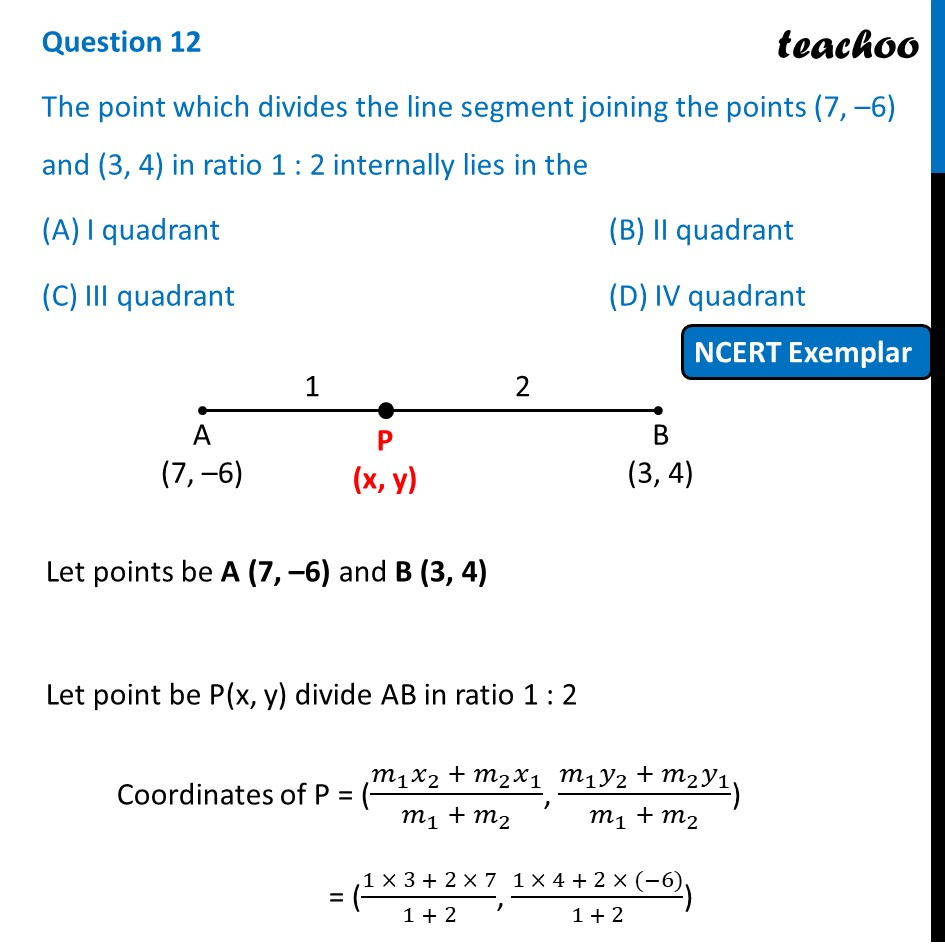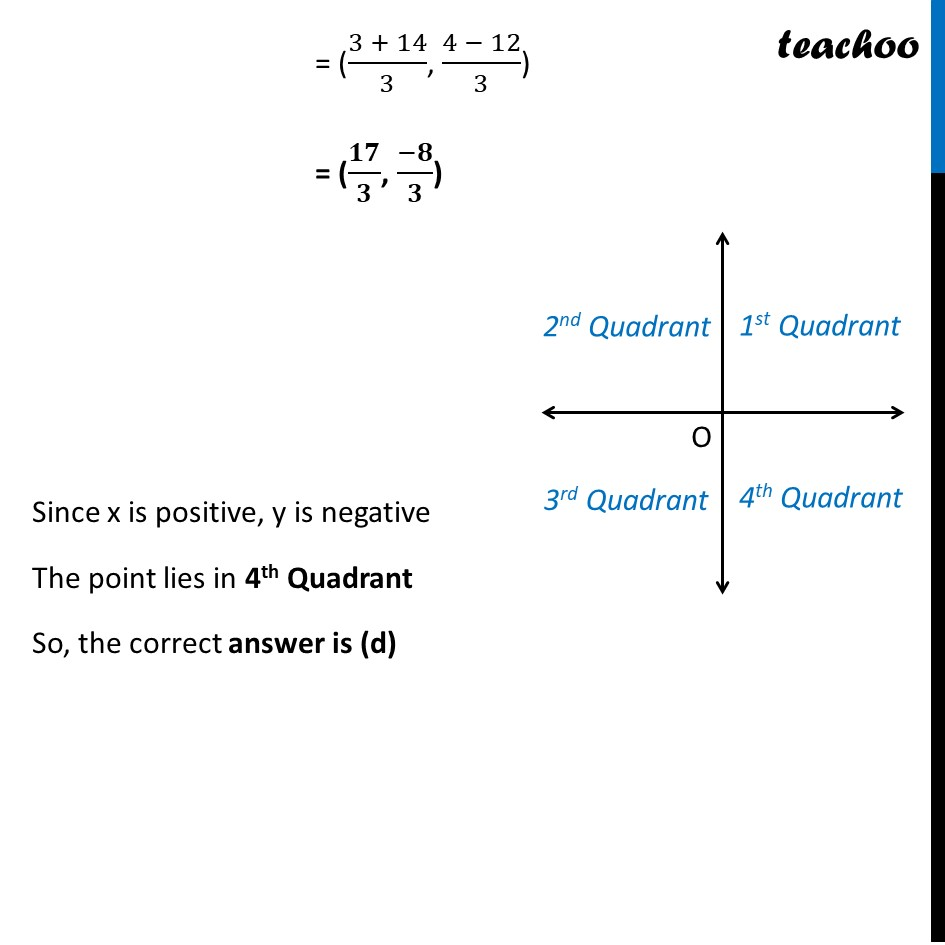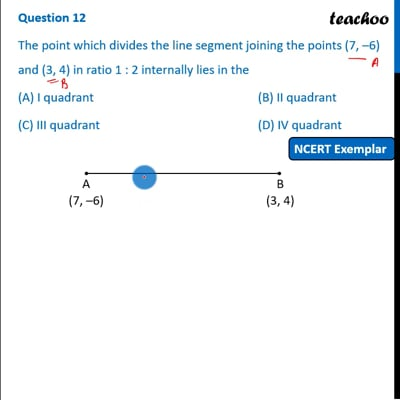NCERT Exemplar - MCQ

Chapter 7 Class 10 Coordinate Geometry
Serial order wise

## The point which divides the line segment joining the points (7, –6) and (3, 4) in ratio 1 : 2 internally lies in the

This question is inspired from  Question 8 - CBSE Class 10 Sample Paper for 2020 Boards - Maths StandardThis video is only available for Teachoo black users

Maths Crash Course - Live lectures + all videos + Real time Doubt solving!

### Transcript

Question 12 The point which divides the line segment joining the points (7, –6) and (3, 4) in ratio 1 : 2 internally lies in the (A) I quadrant (B) II quadrant (C) III quadrant (D) IV quadrant Let points be A (7, –6) and B (3, 4) Let point be P(x, y) divide AB in ratio 1 : 2 Coordinates of P = ((𝑚_1 𝑥_2 + 𝑚_2 𝑥_1)/(𝑚_1 + 𝑚_2 ), (𝑚_1 𝑦_2 + 𝑚_2 𝑦_1)/(𝑚_1 + 𝑚_2 )) = ((1 × 3 + 2 × 7)/(1 + 2), (1 × 4 + 2 × (−6))/(1 + 2)) = ((3 + 14)/3, (4 − 12)/3) = (𝟏𝟕/𝟑, (−𝟖)/𝟑) Since x is positive, y is negative The point lies in 4th Quadrant So, the correct answer is (d)